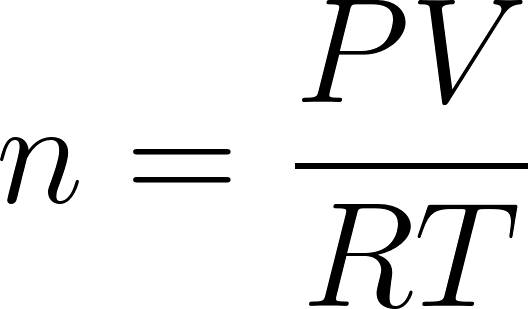×
Get Full Access to Introductory Chemistry - 5 Edition - Chapter 11 - Problem 69p
Get Full Access to Introductory Chemistry - 5 Edition - Chapter 11 - Problem 69p

×

# Solved: An experiment shows that a 248-mL gas sample has a mass of 0.433 g at a pressureISBN: 9780321910295 34

## Solution for problem 69P Chapter 11

Introductory Chemistry | 5th Edition

• Textbook Solutions
• 2901 Step-by-step solutions solved by professors and subject experts
• Get 24/7 help from StudySoup virtual teaching assistantsIntroductory Chemistry | 5th Edition

4 5 1 259 Reviews
30
5
Problem 69P

PROBLEM 69P

An experiment shows that a 248-mL gas sample has a mass of 0.433 g at a pressure of 745 mm Hg and a temperature of 28 °C. What is the molar mass of the gas?

Step-by-Step Solution:

Problem 69P

An experiment shows that a 248-mL gas sample has a mass of 0.433 g at a pressure of 745 mm Hg and a temperature of 28 °C. What is the molar mass of the gas?

Step by Step Solution

Step 1 of 3

According to the ideal gas equation is as follows........................(1)

Rearrange the equation (1) as follows......................(2)

n= Number of moles of the gas

P = Pressure of the gas

V = Volume of the gas

R = Gas constant

T = Temperature of the gas.

Step 2 of 3

Step 3 of 3

##### ISBN: 9780321910295

Unlock Textbook Solution